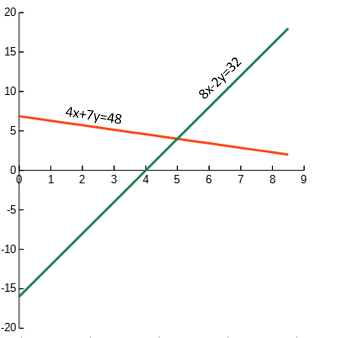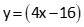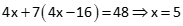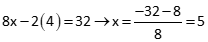## 1. Linear Equations

### a. The Straight and Narrow

A linear equation is a straight line in two or more dimensions. A single equation consists of coefficients, variables, and a constant. In the equation 1.5x-4.5y = 25

x and y are variables.
1.5 and -4.5 are coefficients, numeric values by which the variables are multiplied.
25 is a constant, the result of applying the coefficients to specific variable values.

In a single equation with two variables, assigning a value to either variable defines the other. If both are unknown, the equation cannot be uniquely solved because there are an infinite number of value combinations for x and y that satisfy the equation.

Values of the same two variables in two different linear equations can be determined, providing the equations do not represent parallel lines. There is a single set of values which satisfy both equations at the same time. In graphic terms, x and y are coordinates where both lines intersect.We could solve these equations graphically by plotting both and interpolating their intersection’s coordinates, Figure C-1.Figure C-1 Plotted Equations

Accuracy is limited by our graphing and interpolation abilities

### b. Numeric Solution

The coordinates can be determined with greater accuracy from the equations. There are several ways to do this, two of which are Substitution and Gaussian Elimination.

#### (1) Substitution

Rearrange the first equation in terms of  y.Substitute it into the second equation and solve for x.Then use x to solve for y.#### (2) Gaussian Elimination

One equation is multiplied or divided by a constant, then added to or subtracted from another equation to eliminate one of the variables. The more variables, the more times this is done, creating intermediate equations with fewer variables until there is a single equation in a single variable. The variable is solved and then substituted back into intermediate equations  until all variables are solved. It sounds more complicated than it is.

In this case, multiply the second equation by 2, subtract from the first. This eliminates x and leaves a single equation in terms of y. Solve y.Then substitute y into either equation to solve x.Neither way is particularly difficult, but as the number of equations and unknowns increase (3 equations in 3 unknowns, 4 in 4, etc), the math involved increases accordingly.

Solving simultaneous linear equations is relatively straightforward: their geometry is simple and there is just one intersection point. The only condition the two equations must meet is that they cannot represent parallel lines.